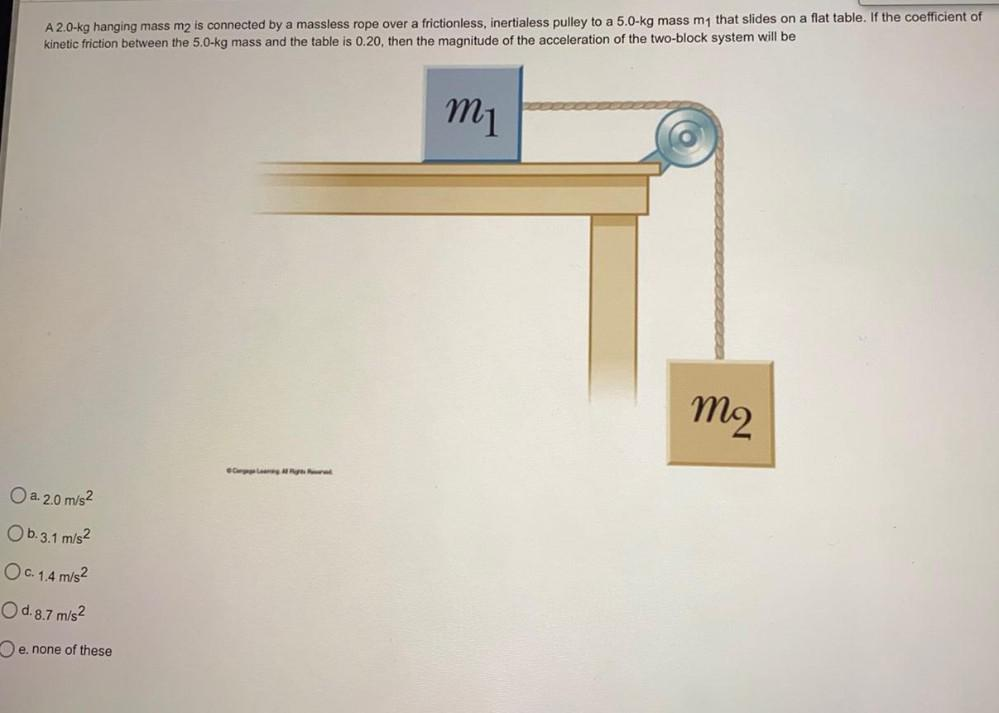Question:

# A 2.0-kg hanging mass m2 is connected by a massless rope over a frictionless, inertialess pulley to a 5.0-kg mass m1 that slidesA 2.0-kg hanging mass m2 is connected by a massless rope over a frictionless, inertialess pulley to a 5.0-kg mass m1 that slides on a flat table. If the coefficient of kinetic friction between the 5.0-kg mass and the table is 0.20, then the magnitude of the acceleration of the two-block system will be M1 m2 Crong at O a. 2.0 m/s2 Ob.3.1 m/s2 O c. 1.4 m/s2 O d. 8.7 m/s2 e. none of these